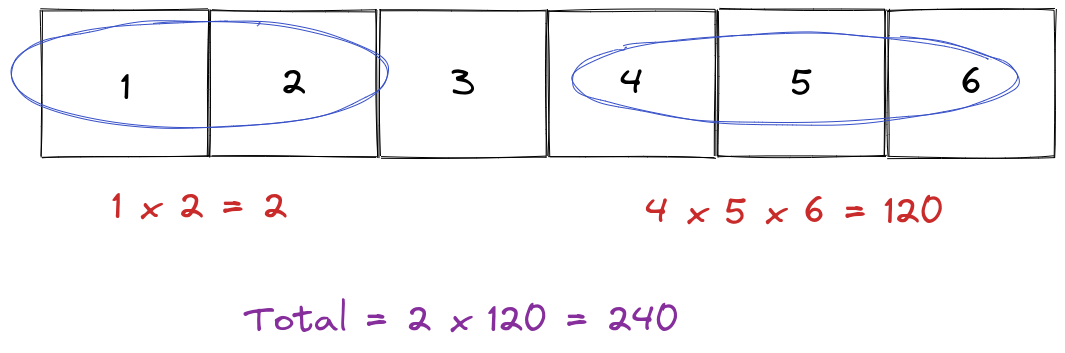Pankaj Tanwar
Published on

# Product of Array Except Self.

––– views
Authors
•Name
Pankaj Tanwar
@the2ndfloorguy

Problem of the day - Product of Array Except Self

Tag - Medium

Given an integer array nums, return an array answer such that answer[i] is equal to the product of all the elements of nums except nums[i].

The product of any prefix or suffix of nums is guaranteed to fit in a 32-bit integer.

You must write an algorithm that runs in O(n) time and without using the division operation.

Example 1:

Input: nums = [1,2,3,4] Output: [24,12,8,6]

I started jumping after looking at the problem. Just calculate the total product of all elements, and divide the current to get the product of the array except self. Pretty easy peasy! WTH this is under the medium tag?

Oh wait, I missed one scary statement.

You must write an algorithm that runs in O(n) time and without using the division operation.

Nightmare! Well, no worries let's find out some optimized way of solving it.

I tried to think of some approach but every approach, either came back to using division operator (which leetcode won't like for this problem) or more than O(n) time complexity.

I thought of reading the problem description again!

Wait for a second, why leetcode has mentioned -

The product of any prefix or suffix of nums is guaranteed to fit in a 32-bit integer.

Where am I using prefix or suffix? Shall I use it somehow to find the algorithm?

Brainstorming again started. And yessssssss! I found the solution.

So, the product of an array except self is the product of prefix and suffix elements.

### Let's understand with below example.

Here, product of array except 3 is -That was pretty clever man!

class Solution {public:    vector<int> productExceptSelf(vector<int>& nums) {        vector<int> res;        int prefix = 1;        for(int i=0;i<nums.size();i++) {            res.push_back(prefix);            prefix *= nums[i];        }                prefix = 1;        for(int i=nums.size()-1;i>=0;i--) {            res[i] *= prefix;            prefix *= nums[i];        }        return res;    }};

### The Algorithm

• We need two passes here
• In the first pass, we start from the beginning of the array, calculate the prefix of each element, and put it in the result vector
• In the second pass, we start from the end of the array, calculate the suffix of each element and multiply it with the existing value in the result vector
• We have the final result

Here, we are matching all requirements, O(n) run time with no extra space & without using division operation.

Quite interesting problem after a long time. See you guys tomorrow!

I welcome your suggestions to improve it further!

Thanks for being part of my daily-code-workout journey. As always, if you have any thoughts about anything shared above, don't hesitate to reach out.

You might like previous editions of my coding diary